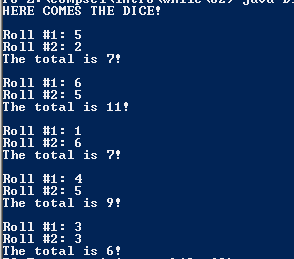# Assignment #62 and Dice Doubles

## Code

```    ///Name: Tim Gibson
///Period: 6
///Project Name: Dice Doubles
///File Name: Dice2.java
///Date Finished: 10/19/15

import java.util.Random;

public class Dice2
{

public static void main ( String[] args )
{

Random r = new Random();

int a, b, c;

a = 1 + r.nextInt(6);
b = 1 + r.nextInt(6);
c = a + b;

System.out.println( "HERE COMES THE DICE!");

System.out.println( "\nRoll #1: " + a + "\nRoll #2: " + b + "\nThe total is " + c + "!" );

while ( a != b )
{
a = 1 + r.nextInt(6);
b = 1 + r.nextInt(6);
c = a + b;
System.out.println( "\nRoll #1: " + a + "\nRoll #2: " + b + "\nThe total is " + c + "!" );
}

}
}
```

### Picture of the output